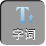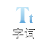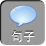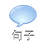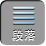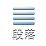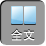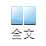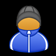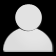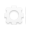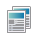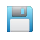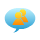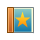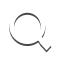-AA+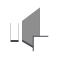“遇斜化直”解“双直角三角形”

“遇斜化直”解“双直角三角形”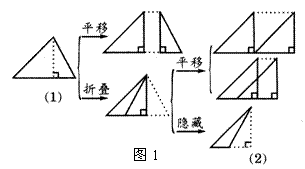1.如图 2，某海监船向正西方向航行，在 A处望见一艘正在作业渔船 D在南偏西 45°方向，海监船航行到 B处时望见渔船 D在南偏东 45°方向，又航行了半小时到达 C处，望见渔船 D在南偏东 60°方向，若海监船的速度为 50海里/时，则 AB之间的距离为（取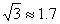，结果精确到 0.1海里）．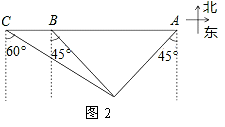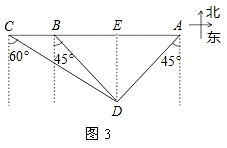DE= x，则 AB= 2 x.

RtCDE中，∠ DCE= 30°，则 CE=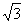DE=x.

RtBDE中，∠ DAE= 45°，则 DE= BE= x.

AB= 25+ 1）= 67.5（海里）．

2. 如图 4，一条自西向东的观光大道 l上有 AB两个景点， AB相距 2 km，在 A处测得另一景点 C位于点 A的北偏东 60°方向，在 B处测得景点 C位于景点 B的北偏东 45°方向，求景点 C到观光大道 l的距离（结果精确到 0.1 km）．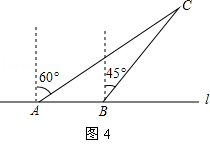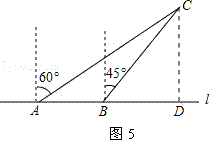AD=CD=xkm

BD= CD= xkm

ADBD= AB，∴xx= 2

x=+ 12.7km）．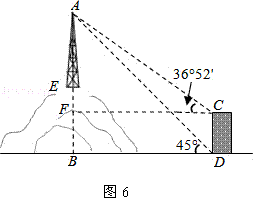RtAFC中，∠ ACF= 36° 52′， AF=（ x+ 29），

CF=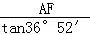=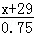= x+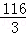RtABD中，∠ ADB= 45°， AB= x+ 56

BD= AB= x+ 56，∵ CF= BD，∴ x+ 56= x+，解得： x= 52